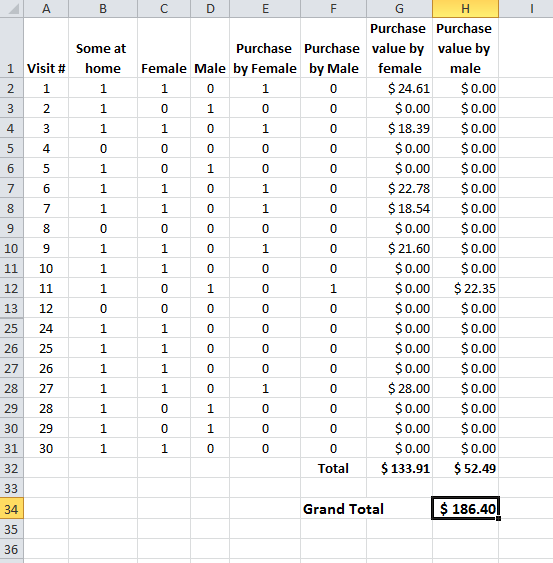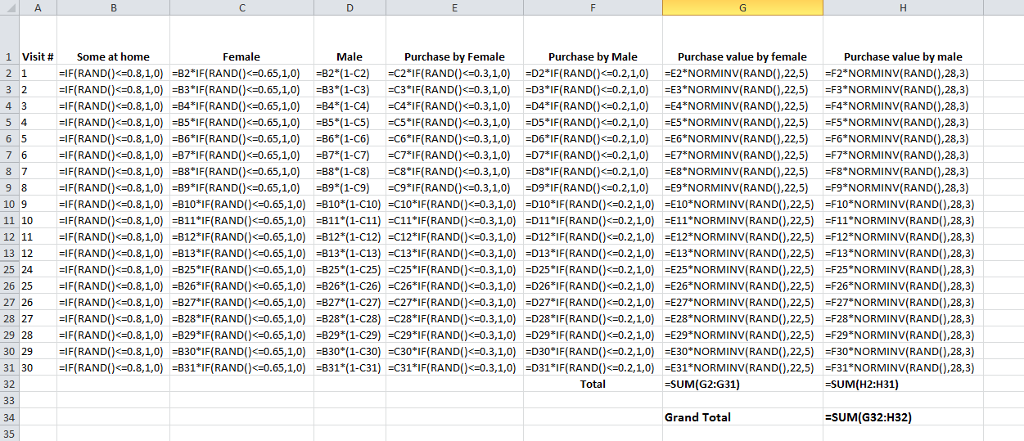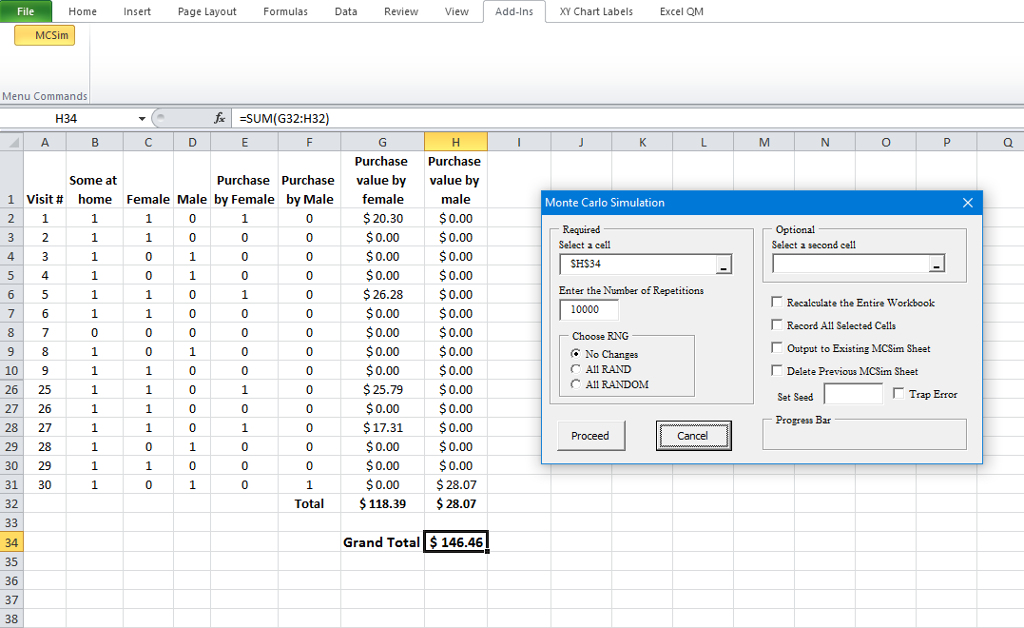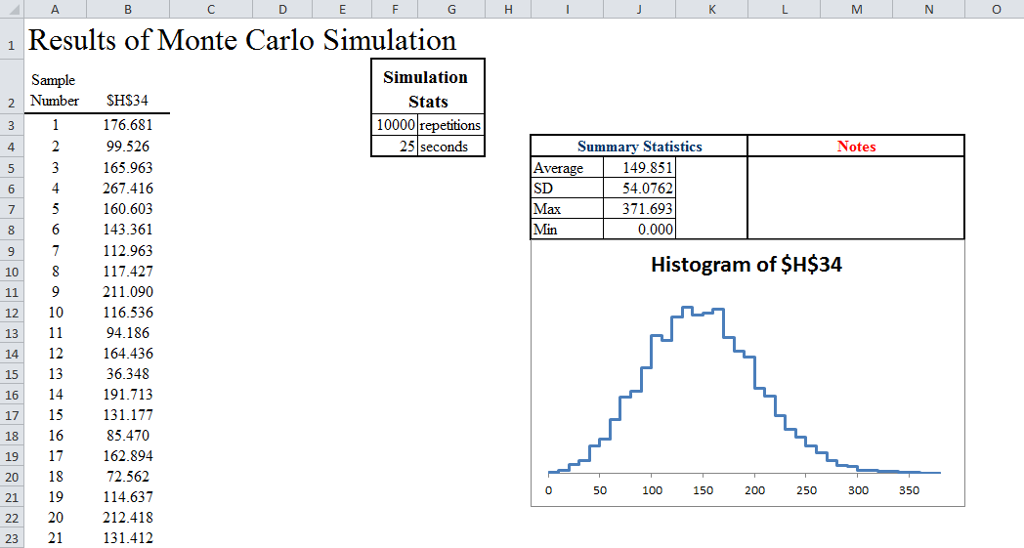# Answered! 2.) Use Microsoft Excel's Analytic Solver Platform to solve the question below:…

2.) Use Microsoft Excel’s Analytic Solver Platform to solve the question below:

An organization is performing a door-to-door marketing campaign to sell goods to consumers. They will visit 30 homes. Consultants estimated they expect to find someone home 80% of the time. When someone is home 65% it is a female. 30% of females make a purchase, and when they make a purchase it is normally distributed with a mean of \$22 and a standard deviation of \$5. 20% of males make a purcahse, and when they do it is normall distributed with a mean of \$28 and a standard deviation of \$3.

Don't use plagiarized sources. Get Your Custom Essay on
Answered! 2.) Use Microsoft Excel's Analytic Solver Platform to solve the question below:…
GET AN ESSAY WRITTEN FOR YOU FROM AS LOW AS \$13/PAGE

1.) What is the total amount they can expect to generate in revenues from these 30 visits?

2.) What is the standard deviation of total revenues over 30 visits?

3.) What is the probability they will make more than 100?

1) Create a Excel spreadsheet model as follows

The spreadsheet model has 30 rows one for each of the 30 visits. Note in the below screenshot, i have hidden some rows for visual convenience. However the formulas in all the rows are same as in the first row.Monte Carlo analytical solver is used for 10000 simulations.Simulation results are as followsFrom the Monte Carlo simulation results above, we see that Average expected revenue = \$ 149.851

refer the summary statistics just above the Histogram.

2) Standard deviation of total revenues over 30 visits = 54.0762

3) Probability that they will make more than \$ 100 correspond to z-value = (100 – 149.851)/54.0762 = – 0.92187

Probability that they will make more than \$ 100 = 1 – NORMSDIST(-0.92187) = 0.8217 or 82.17%